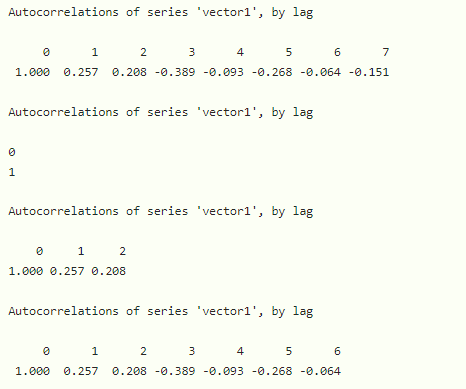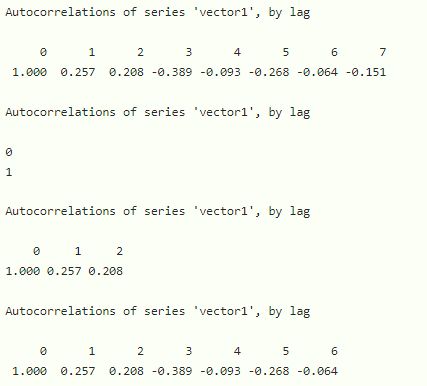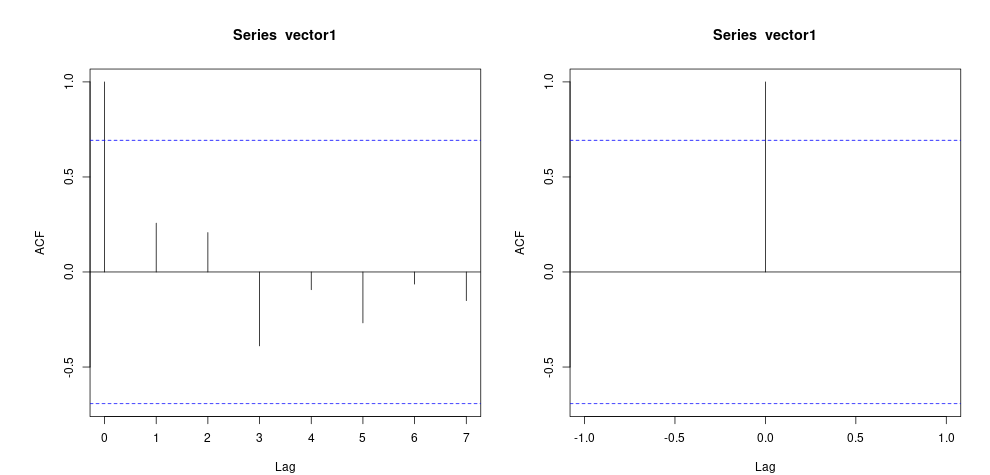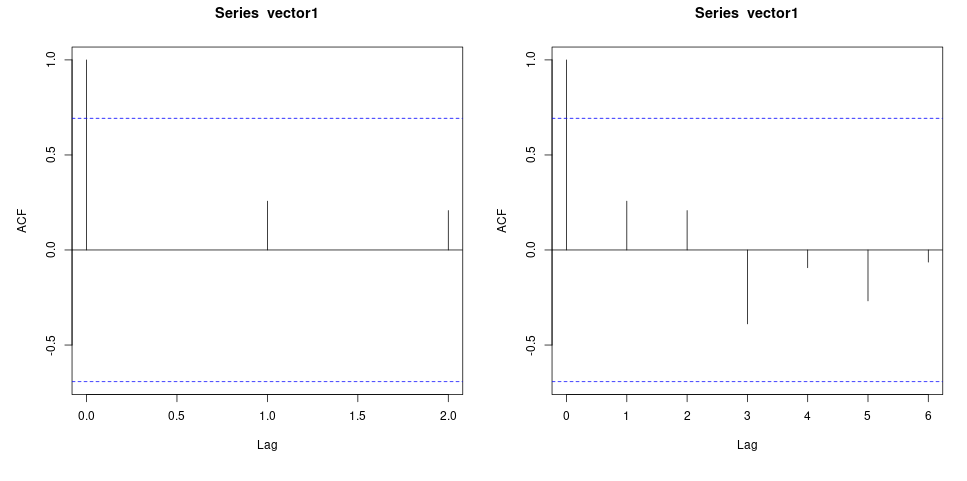# How to Calculate Autocorrelation in R?

• Last Updated : 19 Dec, 2021

Autocorrelation is used to measure the degree of similarity between a time series and a lagged version of itself over the given range of time intervals. We can also call autocorrelation as  “serial correlation” or “lagged correlation”. It is mainly used to measure the relationship between the actual values and the previous values.

In R, we can calculate the autocorrelation in a vector by using the module tseries. Within this module, we have to use acf() method to calculate autocorrelation.

Syntax:

acf(vector, lag, pl)

Parameter:

• vector is the input vector
• lag represents the number of lags
• pl is to plot the auto correlation

Example: R program to calculate auto correlation in a vector with different lags

## R

 `# load tseries module ` `library``(tseries) ` ` `  `# create vector1 with 8 time periods ` `vector1=``c``(34,56,23,45,21,64,78,90) ` ` `  `# calculate auto correlation with no lag ` `print``(``acf``(vector1,pl=``FALSE``)) ` ` `  `# calculate auto correlation with lag 0 ` `print``(``acf``(vector1,lag=0,pl=``FALSE``)) ` ` `  `# calculate auto correlation with lag 2 ` `print``(``acf``(vector1,lag=2,pl=``FALSE``)) ` ` `  `# calculate auto correlation with lag 6 ` `print``(``acf``(vector1,lag=6,pl=``FALSE``)) `

Output:The same function can be used to visualize the output produced for that we simply have to set pl to TRUE

Example: Data visualization

## R

 `# load tseries module ` `library``(tseries) ` ` `  `# create vector1 with 8 time periods ` `vector1=``c``(34,56,23,45,21,64,78,90) ` ` `  `# calculate auto correlation with no lag ` `print``(``acf``(vector1,pl=``TRUE``)) ` ` `  `# calculate auto correlation with lag 0 ` `print``(``acf``(vector1,lag=0,pl=``TRUE``)) ` ` `  `# calculate auto correlation with lag 2 ` `print``(``acf``(vector1,lag=2,pl=``TRUE``)) ` ` `  `# calculate auto correlation with lag 6 ` `print``(``acf``(vector1,lag=6,pl=``TRUE``)) `

Output:My Personal Notes arrow_drop_up
Recommended Articles
Page :